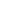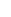# How the three kinds of bending angles are unfolded!

Views: 38     Author: Durmapress     Publish Time: 2020-05-07      Origin: Durmapress1. Press Brake to process Small V fold large angle, large V pressurization

When the internal bending workpiece shape is limited, this method is used for processing <as shown below>For a small amount and high processing shape requirements, this processing method is adopted, <as shown below>

a. It is better to choose the thickness of the gasket to process the plate thickness,

b. When selecting V-groove, calculate with two plate thicknesses3. When the round hole is bent and pulled, it can be processed by reaming before bending

As shown below:4. When the square hole is bent and pulled, the secondary processing of the simple mold can be used.

As shown below:5. Special processing of L-folding: <L <V / 2> adopts feeding and bending, and the excess material is removed

after bending (secondary processing) as shown in the figure below:6. Packing bending:

Outer package material bending and inner package material bending, (as shown below)

Cushion grooves should be opened at the corners of the package materials so that there is no extrusion deformation after processing.

Inner package bending = => Bending first, A, B then bending C

Outsourcing material bending = => First bend C, then bend A, BConclusion :

For Example :

How to calculate the expansion of sheet metal bending at a large angle. Fold an angle of 135 degrees, the length of both sides are 60 and 30. The thickness of the plate is 3.0, how to calculate his expansion

1. L = the sum of the inner or outer layer length

"

2. L = A + B- (180-a) * Bending factor / 90 "This is the angle

3. L = A + B-1 / 2 * plate thickness This is calculated as the dead edge

5. L = A + B_K = A + B-2T + π (R2-T + T / 3) / 2 This is a large R angle

6. L = A + B + C-plate thickness, keep a little0086 555 8327689+86 18325572889info@durmapress.com